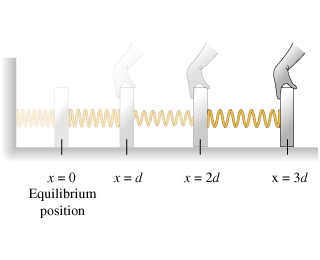# Problem: During which interval is the largest amount of energy required to stretch the spring?a) From x = 0 to x = db) From x = d to x = 2dc) From x = 2d to x = 3dd) The energy required is the same in all three intervals

###### FREE Expert Solution

Potential energy:

$\overline{){\mathbf{P}}{\mathbf{E}}{\mathbf{=}}\frac{\mathbf{1}}{\mathbf{2}}{\mathbf{k}}{{\mathbf{x}}}^{{\mathbf{2}}}}$

The energy required, E = ΔPE

$\overline{){\mathbf{E}}{\mathbf{=}}\frac{\mathbf{1}}{\mathbf{2}}{\mathbf{k}}{\mathbf{\left(}}{{\mathbf{d}}}^{{\mathbf{2}}}{\mathbf{-}}{{\mathbf{x}}}^{{\mathbf{2}}}{\mathbf{\right)}}}$

a)

E = (1/2)k(d2 - 02) = (1/2)kd2

87% (228 ratings)###### Problem DetailsDuring which interval is the largest amount of energy required to stretch the spring?

a) From x = 0 to x = d

b) From x = d to x = 2d

c) From x = 2d to x = 3d

d) The energy required is the same in all three intervals